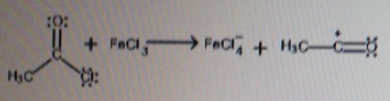# Problem: For the following reaction, indicate which reactant is the Lewis acid and which is the Lewis base.a) CH3COCl is the Lewis acid.b) CH3COCl is the Lewis base.c) FeCl3 is the Lewis acid.d) FeCl3 is the Lewis base.e) This not a Lewis acid-base reaction.

###### FREE Expert Solution

Based on the Lewis definition:

Lewis acid is an electron pair acceptor.

Some characteristics of a Lewis acid:

When hydrogen is connected to an electronegative element such as P, O, N, S or halogens

▪ hydrogen gains a partially positive charge → makes hydrogen act as a Lewis acid

Positively charged metal ions

97% (142 ratings)###### Problem Details

For the following reaction, indicate which reactant is the Lewis acid and which is the Lewis base.a) CH3COCl is the Lewis acid.

b) CH3COCl is the Lewis base.

c) FeCl3 is the Lewis acid.

d) FeCl3 is the Lewis base.

e) This not a Lewis acid-base reaction.